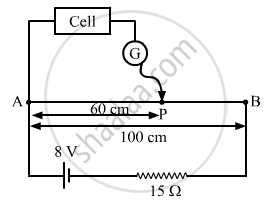# A Potentiometer Wire of Length 1 M Has a Resistance of 5 ω. It is Connected to a 8 V Battery in Series with a Resistance of 15 ω. Determine the Emf of the Primary Cell Which Gives a Bala - Physics

A potentiometer wire of length 1 m has a resistance of 5 Ω. It is connected to a 8 V battery in series with a resistance of 15 Ω. Determine the emf of the primary cell which gives a balance point at 60 cm.

#### SolutionFrom the figure:
Total resistance of the circuit, R = (RAB + 15) Ω = 20 Ω
Current in the circuit ,

$i = \frac{V}{R} = \frac{8}{20} A$

∴ Voltage across ABVAB = i.RAB = 2 V
The emf of the cell connected as above is given by: $e = \frac{l}{L} V_0$

Here:
l = 60 cm (balance point)
AB = L = 1 m = 100 cm (total length of the wire)

$\therefore e = \frac{60}{100}\left( 2 \right) = 1 . 2 V$

Concept: Potentiometer
Is there an error in this question or solution?